####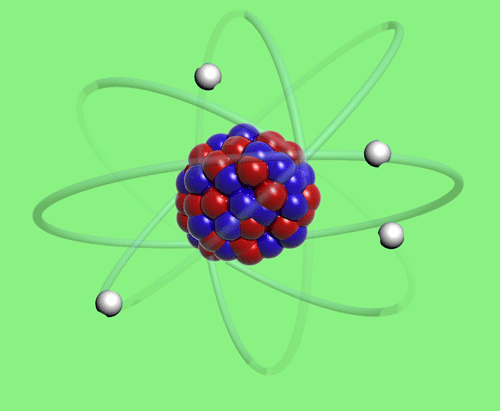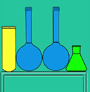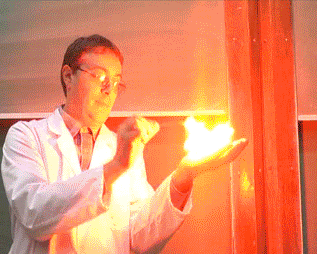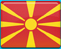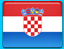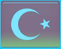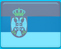## Determination of the Oxygen Content in the Air

A simple and fast determination of oxygen content in the air is proposed based on the reaction between NO(g) and oxygen.

2NO(g) + O2(g) = 2NO2(g)                                 (1)

In the experiment NO(g) is first produced by the reaction of iron(II) ions with nitrogen dioxyde-(equation 2) and after that, we react it with air (oxygen). The nitrogen dioxyde that is produced in this process is soluble in water and reacts with it (equation 3).

Fe2+(aq) + NO2-(aq) + 2H+(aq) = Fe3+(aq) + NO(g) + H2O(l)                (2)

2NO2(aq) + H2O(l) = HNO3(aq) + HNO2(aq)                         (3)

Reaction 1 is over, only nitrogen and trace gases from air are left (as gases) in the system.  From volume measurements at the beginning and the end of the reaction, the oxygen content of the air can be determined.

Materials

Liquemin ampoule (5 mL), stand, shortened Beral pipet, two 1 mL and one 5 mL syringes, permanent marker, saturated solution of sodium nitrite, solution of iron(II) sulfate (250 mg in 5 mL HCl (15-20 %))

#### Step 1

Collect and check the needed materials.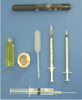#### Step 2

Place the vial with iron(II) sulfate solution on the stand. Open it and completely fill the pipet with the solution.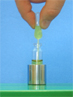Fill one syringe with 1 mL of air and another one with 0,2 mL saturated solution of sodium nitrite.
Insert the needle of the second syringe into the Beral pipet bulb.
Carefully, “drop by drop”, add the solution of sodium nitrite
until one third of the Beral pipet bulb is filled with a
colourless gas (NO).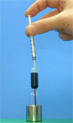Step 4

Mark the liquid level in the pipet bulb with a permanent marker.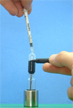Step 5

Insert the needle of another syringe with 1 mL of air
in the Beral pipet head and slowly push the air into the pipet.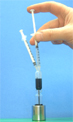Step 6

Hold the two syringes and shake the pipets to provide appropriate conditions for dissolving the generated nitrogen dioxyde.
Do not wait too long.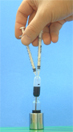Step 7

After that, with the second syringe, suck out a part of the gas, until the liquid in the pipet bulb reaches the marked level.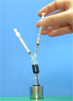Step 8

Read the volume of the gas in the syringe, write it down and make the calculations as explained later.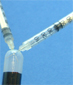Step 9

Eliminate the NO waste by drawing it into a syringe
containing 2 mL water and 2 mL air.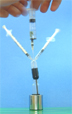Calculation of the oxygen content in the air

oxygen content = Voxygen ·100

Let us mark the unknown volume of NO in the pipet bulb with Vx. This is the marked volume. The volume of air is
1 mL. So the total volume
Vt = Vx + 1 mL
The final volume (Vf) is a sum of the volume of the gas that is set on the mark (that means Vx) and the volume of the gas that is in the syringe which value could be read with the scale on the syringe (Vread):